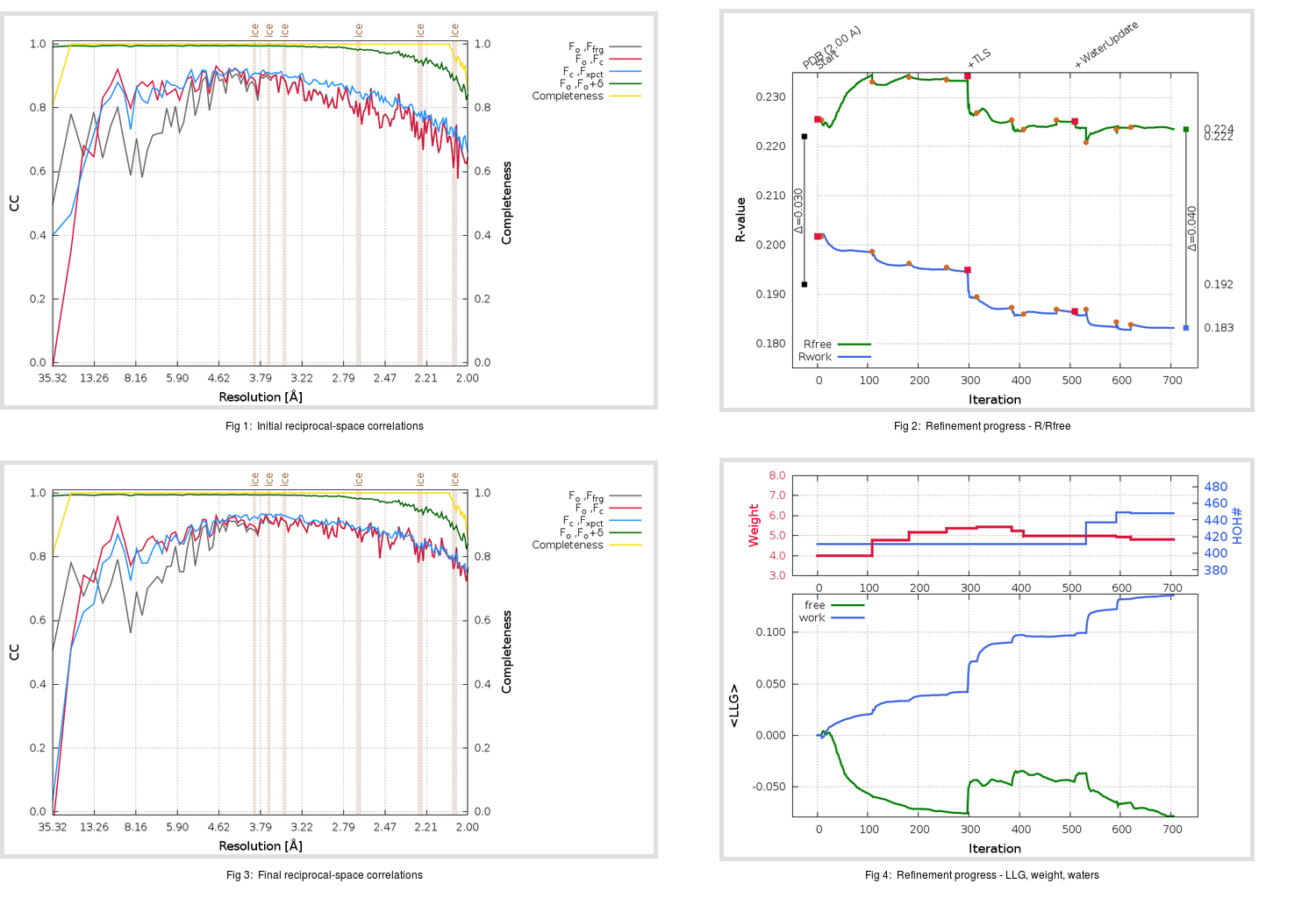Content:

```    Diffraction limits & principal axes of ellipsoid fitted to diffraction cut-off surface:
2.176         1.0000   0.0000   0.0000       a*
1.907         0.0000   1.0000   0.0000       b*
1.974         0.0000   0.0000   1.0000       c*
```

## Deposited

` `
 Date deposited Date data collection Resolution R, Rfree 20170525 20151203 2.00 0.1910 0.2220

Molprobity (CCP4 7.0 version) summary:

```Ramachandran outliers =   0.00 %
favored =  97.35 %
Rotamer outliers      =   0.34 %
C-beta deviations     =     1
Clashscore            =   4.32
RMS(bonds)            =   0.0050
RMS(angles)           =   1.05
MolProbity score      =   1.34
Resolution            =   2.00
R-work                =   0.1910
R-free                =   0.2220
```

```Number of waters      =   411

<B> (all atoms) =   27.77 ( sd =   10.29 ) for       3271 non-hydrogen atoms
<B>   (protein) =   25.65 ( sd =    8.54 ) for       2703 non-hydrogen atoms
<B>     (water) =   35.48 ( sd =   10.68 ) for        411 non-hydrogen atoms
<B>    (others) =   44.00 ( sd =   12.41 ) for        157 non-hydrogen atoms

B min/max       (all non-hydrogen atoms) =    5.55 /   75.33
B min/max   (protein non-hydrogen atoms) =   10.73 /   69.85
B min/max     (water non-hydrogen atoms) =    5.55 /   63.02
B min/max     (other non-hydrogen atoms) =   18.83 /   75.33
```

## BUSTER (re-)refinement

` `

Molprobity (CCP4 7.0 version) summary:

```Ramachandran outliers =   0.00 %
favored =  96.47 %
Rotamer outliers      =   1.03 %
C-beta deviations     =     2
Clashscore            =   1.80
RMS(bonds)            =   0.0120
RMS(angles)           =   1.63
MolProbity score      =   1.18
Resolution            =   2.00
R-work                =   0.1833
R-free                =   0.2236
```

```Number of waters      =   448

<B> (all atoms) =   27.10 ( sd =   10.59 ) for       3308 non-hydrogen atoms
<B>   (protein) =   24.18 ( sd =    7.48 ) for       2703 non-hydrogen atoms
<B>     (water) =   38.23 ( sd =   12.41 ) for        448 non-hydrogen atoms
<B>    (others) =   45.67 ( sd =   10.70 ) for        157 non-hydrogen atoms

B min/max       (all non-hydrogen atoms) =    6.80 /   78.18
B min/max   (protein non-hydrogen atoms) =    6.80 /   56.96
B min/max     (water non-hydrogen atoms) =    7.89 /   78.18
B min/max     (other non-hydrogen atoms) =   23.85 /   62.65
```

Refinement progression:Results:

` `
 File Remark 5VYH_aB_refine.01_03_refine.pdb.gz exact refinement commands are in header 5VYH_aB_refine.01_03_refine.mtz.gz including original deposited data and several re-refinement map coefficients 5VYH_aB_refine.01_03_BUSTER_model.cif.gz including any non-standard compound restraints 5VYH_aB_refine.01_03_BUSTER_refln.cif.gz